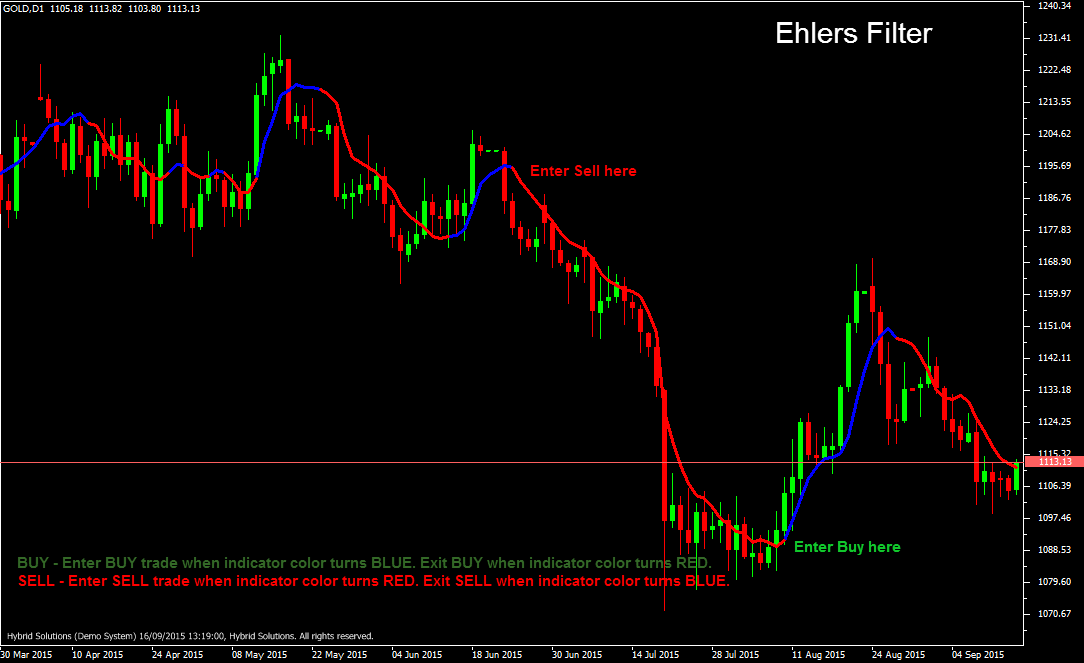# Ehlers Filter

Free

Developed By: NeeduVerdeSolution

In stock

Ehlers Filter is a powerful trend-following VertexFX indicator, which provides precise BUY and SELL signals.This indicator has a minimal lag, of approximately 4 bars, which is very useful in detecting start of trends.

SKU: 689 Categories: ,Ehlers Filter is a powerful trend-following VertexFX indicator, which provides precise BUY and SELL signals.This indicator has a minimal lag, of approximately 4 bars, which is very useful in detecting start of trends. At first we calculate the smoothing coefficient, which is the absolute difference between the current price and price MOM_PERIOD bars ago. Next we smooth the price using this smoothing coefficient over the LENGTH bars period. This is called the Ehlers filter.

The current Ehler filter is based on acceleration and speed. The filter uses the close and volume data along with three inputs/parameters to smooth the price series. The parameters that should be provided to the Ehler filter function are:
– Length of the FIR (Finite Impulse Response). This is one type of filter, the other one is the Infinite Impulse Response (IIR). For example, the EMA is an IIR filter.
– Exponential weight of passed acceleration and speed
– Weighting factor between acceleration and speed

The Ehler filter is interpreted like any moving average where usually a close price above the Ehler filter is considered a bullish sign and a value below the filter is considered a bearish sign.

BUY – Place Buy position when indicator color turns Blue. Exit Buy when indicator color turns Red.
SELL – Place Sell position when indicator color turns Red. Exits Sell when indicator color turns Blue.